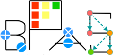Basic Polynomial Algebra Subprograms (BPAS)  v. 1.652
BPASRing< Derived > Class Template Referenceabstract

An abstract class defining the interface of a commutative ring. More...

`#include <BPASRing.hpp>`

Simplified semantic inheritance diagram for BPASRing< Derived >:Full inheritance diagram for BPASRing< Derived >:
[legend]

## Public Member Functions

virtual mpz_class characteristic ()
The characteristic of this ring class.

virtual bool isZero () const =0
Determine if *this ring element is zero, that is the additive identity. More...

virtual void zero ()=0
Make *this ring element zero.

virtual bool isOne () const =0
Determine if *this ring element is one, that is the multiplication identity. More...

virtual void one ()=0
Make *this ring element one.

virtual Derived unitCanonical (Derived *u=NULL, Derived *v=NULL) const =0
Obtain the unit normal (a.k.a canonical associate) of an element. More...

virtual Derived & operator= (const Derived &)=0
Copy assignment.

virtual Derived operator+ (const Derived &) const =0
Addition.

virtual Derived & operator+= (const Derived &)=0
Addition assignment.

virtual Derived operator- (const Derived &) const =0
Subtraction.

virtual Derived & operator-= (const Derived &)=0
Subtraction assignment.

virtual Derived operator- () const =0
Negation.

virtual Derived operator* (const Derived &) const =0
Multiplication.

virtual Derived & operator*= (const Derived &)=0
Multiplication assignment.

virtual Derived operator^ (long long int e) const =0
Exponentiation.

virtual Derived & operator^= (long long int e)=0
Exponentiation assignment.

virtual bool operator== (const Derived &) const =0
Equality test,. More...

virtual bool operator!= (const Derived &) const =0
Inequality test,. More...

virtual void print (std::ostream &ostream) const
Print the Ring element. More...Public Member Functions inherited from ExpressionTreeConvert
virtual ExpressionTree convertToExpressionTree () const =0
Convert this to an expression tree. More...

## Static Public Attributes

static RingProperties properties
Static element describing the properties of this ring class.

## Friends

std::ostream & operator<< (std::ostream &ostream, const Derived &d)
Output operator. More...

std::ostream & operator<< (std::ostream &ostream, Derived &&d)

## Detailed Description

### template<class Derived> class BPASRing< Derived >

An abstract class defining the interface of a commutative ring.

The template Derived is a concrete class derived from (implemeneting the interface of) BPASRing. This is the "curiously recurring template pattern" (CTRP). This pattern is used among all sub-classes of BPASRing.

## ◆ isOne()

template<class Derived>
 virtual bool BPASRing< Derived >::isOne ( ) const
pure virtual

Determine if *this ring element is one, that is the multiplication identity.

returns true iff *this is one.

## ◆ isZero()

template<class Derived>
 virtual bool BPASRing< Derived >::isZero ( ) const
pure virtual

Determine if *this ring element is zero, that is the additive identity.

returns true iff *this is zero.

## ◆ operator!=()

template<class Derived>
 virtual bool BPASRing< Derived >::operator!= ( const Derived & ) const
pure virtual

Inequality test,.

returns true iff not equal.

## ◆ operator==()

template<class Derived>
 virtual bool BPASRing< Derived >::operator== ( const Derived & ) const
pure virtual

## ◆ print()

template<class Derived>
 virtual void BPASRing< Derived >::print ( std::ostream & ostream ) const
inlinevirtual

Print the Ring element.

Derived classes may override this to get custom printing that may be more expressive (and prettier) than expression tree printing.

## ◆ unitCanonical()

template<class Derived>
 virtual Derived BPASRing< Derived >::unitCanonical ( Derived * u = `NULL`, Derived * v = `NULL` ) const
pure virtual

Obtain the unit normal (a.k.a canonical associate) of an element.

If either parameters u, v, are non-NULL then the units are returned such that b = ua, v = u^-1. Where b is the unit normal of a, and is the returned value.

## ◆ operator<<

template<class Derived>
 std::ostream& operator<< ( std::ostream & ostream, const Derived & d )
friend

Output operator.

Defines a to string conversion.

The documentation for this class was generated from the following file: